IF IT'S EDUCATION, IT'S ITS
Pathways To Learning... Since 2005
Hong Kong Registered School 566985

# T - tan to two-way classification - Mathematics Dictionary

A   B   C   D   E   F   G   H   I   J   K   L   M   N   O   P   Q   R   S   T   U   V   W   X   Y   Z

tan: A shorthand for the trigonometric function tangent.

tangency: The condition of being tangent to a geometric object or the extended algebraic definition related to the geometric idea of tangents.

tangent: 1. A line which touches a curve locally at only one point, in the same direction (instantaneously) as the curve..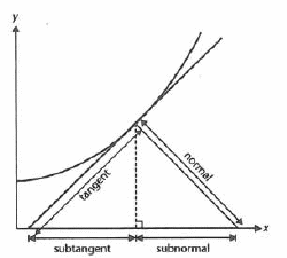2. One of the trigonometric functions , it is the length between the point of contact and intersection between the x-axis and the tangent to a unit circle centre at the origin, where the radius (from the point of contact) makes a specified angle from the positive x-axis.

tangent curve: A graphical representation of the trigonometric function of tangent.tangent formulae: The compund and double angle formulae etc., which is related to the trigonometric function of tangent.

tangential: 1. Being a tangent (to another object/at a given point).

2. Being in the same direction as the tangent (to another object/at a given point).

tangent-secant theorem: One of the circle theorems, it can be seen as a limiting case of the intersecting secant theorem (outside the circle). It states that the product of the distances from the intersections of a secant and the circumference to the intersection of the secant and the tangent, is the square of the distance from the intersection of the secant and the tangent to the contact point of the tangent.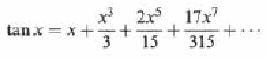tanh: A shorthand for the hyperbolic function of hyperbolic tangent.

tautochrone: Also known as an isochrone

Taylor series remainder: A truncated Taylor series, where a finite number of terms is deleted from the beginning (so that if a term is deleted, all the terms prior to it are also deleted). It is the difference between the function and its Taylor Series approximation (by only evaluating up to a certain term).

Taylors theorem: The representation (or approximation) of a specified function by a power series where coefficients are functions of the derivatives of the specified function over a specific point.

t-distribution: Also known as student's t-distribution. It is the distirbution of the population mean, given the sample mean and the sample (not population) variance.

tend to: A sequence/function tends to a value L if L is the limit of the sequence/function.

tension: The magnitude of a force applied by an object along its length towards the force applying object as a reaction to another force or in order to return to its natural shape.

term: An expression (usually within a larger expression) which is considered as one, connected by most elementary operations and functions other than addition and subtraction.

terminal speed: The speed at which an object free-falling under gravity achieves (or tends towards) as a result of the equilibrium between gravity (which is constant) and air-resistance (which is proportional to the square of speed).

terminating decimal: A recurring decimal where 0 is the digit which even recurrs (regularly at every digit). Thus, a terminating decimal is (just like a recurring decimal) a rational number.

terminating fraction: A continued fraction which is finite.

tessellation: A pattern where polytopes are placed in a space so that the geometric figures do not overlap and no space is not covered for an arbitrarily large space.

tetrahedron: A pyramid with a triangular base. A solid figure with 4 vertices, 6 edges and 4 (triangular) faces.

theorem: A statement which has been proven true by sentences already established to be true: i.e. either axioms (assumed to be true) or other theorems

three-body problem: The problem of providing a general method for detemining all subsequent motions of 3 objects, interacting through only gravity, from all initial conditions (of mass, position and velocity).

three dimensions: Space which must be labelled by 3 separate numbers (coordinates) so that closer points have closer coordinates and closer coordinates implies closer points.

tiling: Tessellation in 2 dimensions.

time dilation: A physical phenomenon described by the theory of relativity where the times between the same 2 events are measured to be different by two observers, at different velocities or in different frames of reference.

ton: 1. A British unit of weight equals to the force of 2240 pounds. Also known as the long ton.

2. A North American unit of weight equals to the force of 2000 pounds.

tonne: A metric unit of mass of 1000 kilograms. It is sometimes called metric tonne to verbally distinguish it from the ton

torque: Also known as angular moments. It is the influence of a force on the rotation of a system. It is the product of the magnitude of the force and the perpendicular distance between the force and a point from which the influence (moment) is measured.

total differential: An exact differential.

totient function: The function whose value is the number of totitives of the argument, which is another positive integer.

totitive: Mostly spelled totative in modern times, a positive integer which is coprime to and less than a specified number. The number 1 is considered to be a totitive of all positive integers.

tower: A countable category of objects, indexed by the natural numbers, such that morphisms go (and only go) from higher-indexed objects to lower-indexed objects.

trace: The sum of the elements in the leading diagonal of a matrix.

trail: A walk where no edges are repeated.

trajectory: The curve traced by the an object in motion.

transcendental function: A function which is the root of a polynomial of 2 variables (where the function substitutes into one of the variables) with integer coefficients.

transcendental number: A real number which is not an algebraic number.

transformation: 1. Any general methods representing a mathematical function (of a certain type) in a different form (usually for a particular purpose).

2. A function from a space to itself.

transformation of axes: Describing vectors in the same type of coordinate system, but with different references axes.

transitive relation: A relation R is transitive if xRy and yRz together implies that xRz for all elements x, y and z.

translation: A transformation where all points in space is moved along the same (non-zero) vector (same direction and magnitude). Specifically, with no invariant points.

translation of axes: A transformation of axes where the new direction of each of the axes is parallel to the (corresponding) old direction.

transpose: A matrix where the rows and columns are swapped from another matrix. Given a matrix M with elements mi,j the transpose of M, MT = N such that ni,j = mj,i

transversal: A line intersecting two or more other lines in the same plane.

transverse axis: The axis of symmetry of a hyperbola which intersects the hyperbola.

trapezium: A quadrilateral with exactly one pair of parallel sides. Known as a trapezoid in the US.

trapezoidal rule: Also known as the trapezium rule, it is a method for estimating the value of a definite integral by dividing the area under the curve into a finite number of trapeziums (trapezoids).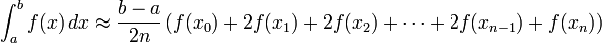with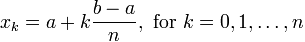travelling salesman problem: The problem of finding the Hamiltonian cycle of the least weight in an undirected, complete, weighted graph in Graph Theory.

tree: A connected, acyclic graph, i.e. a graph with exactly one path between any two vertices.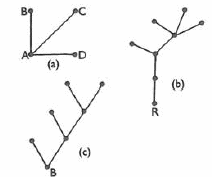tree diagram: A directed tree where no two edges point towards the same vertex, often used in representing events in probability calculationstrend line: A line used to represent values while ignoring small fluctuations.

trial: An experiment within a series of experiments.

triangle: A polygon of 3 sides.

Triangles can be classified by the relative lengths of the 3 sides:

• A triangle of 3 equal sides is called an equilateral triangle. (In the case of triangles, an equilateral triangle is also equiangular, thus regular. The use of equilateral triangle and not equiangular and regular is a convention.)

• A triangle with 2 equal sides is an isosceles triangle.

• A triangle with no equal sides is a scalene triangle.

triangular inequality: The statement that a + b ≥ c for all a, b, c within a set of mathematical objects.

triangular matrix: A non-zero matrix, where the elements on mi,j = 0 for all i > j (lower triangular matrix) or j > i (upper triangular matrix) or i ≥ j (strictly lower triangular matrix) or j ≥ i (strictly upper triangular matrix).

triangular number: Also known as a triangle number. A number which is the sum of all integers not greater than a specified integer.

triangular prism: A prism with a triangular base.

trigonometric functions: Also known as circular functions. Originally, functions defined by a right-angled triangle with one of the non-right-angles as a reference angle such that: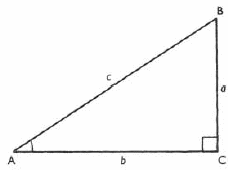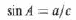where a is known as the opposite side, b the adjacent side and c the hypotenuse.

sin is the shorthand for the elementary trigonometric function sine. cos is the shorthand for the elementary trigonometric function cosine and tan if the shorthand for the elementary trigonometric function tangent.

Such a definition allows for angles between 0o and 90o.

There are also 3 reciprocal trigonometric functions: secant (sec), cosecant (cosec or csc), cotangent (cot), defined as the reciprocal of cosine, sine and tangent, respectively.

trigonometric ratios: The values of the trigonometric functions. A trigonometric function takes angles as arguments and trigonometric ratios as values.

Trigonometry: The geometric study of triangles and algebraic study of trigonometric functions.

trillion: The class="d-title" name variously given to a thousand billion and a million billion in the past. Traditionally, the US used a trillion as the former and the UK as the latter. With the rapid globalisation in the past few decades, the US definition has become more prevalent, even within the UK.

trinomial: A polynomial of three terms.

triple integral: A iterated integral consisting of 3 successive integrations.

truncation: 1. The representation of a number (as a sequence of digits), a sequence or a series by disregarding all digits/terms after a certain digit/term. i.e. in the case of numbers, representing a number up to a certain decimal place by always assuming that the first digit to cut off does not require the rounding up of the last digit presented.

2. An operation on a solid angle of a polytope by retaining only the intersection of the polytope and a half space (i.e. half of the space of dimension n separated by a hyperplane of dimension n-1)

truth table: Tables representing the truth values of propositions in propositional logic.

t-test: Hypothesis testing where the statistic has the t-distribution.

turning point: Also known as a stationary point. A point on the graph of a function where the tangent (or tangent plane) is perpendicular to the axis representing the value (output) of a function.

twin primes: A pair of consecutively odd prime numbers.

twisted curve: An elliptic curve formed from another elliptic curve by adding (amending) coefficients to certain terms.

two-person game: A game consisting of 2 players (decision makers) in game theory.

two-point form: The expression of a line in equations in a form which clear shows 2 of the points through which the line goes.

two-tail test: Also known as a two-tailed test. Hypothesis testing where the alternative hypothesis (H1) is an assertion that the statistic is not of a certain value (specified in H0), thus allowed to be either lower or greater than the specified value.

two-way classification: The classification of a data set by 2 criteria, i.e. representable in a table.

• Type I error is the act of falsely rejecting the null hypothesis (H0) in hypothesis testing when the null hypothesis is true

• Type II error is the act of falsely accepting (or false not rejecting) the null hypothesis (H0) in hypothesis testing when the null hypothesis is false

A   B   C   D   E   F   G   H   I   J   K   L   M   N   O   P   Q   R   S   T   U   V   W   X   Y   Z

Genius is one percent inspiration and ninety-nine percent perspiration.